# Physics 121 Electricity Magnetism Lecture 11 Induction I

• Slides: 18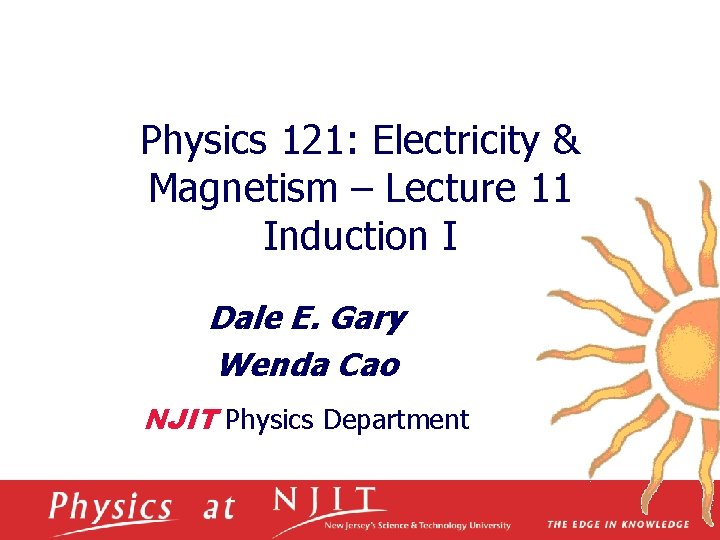Physics 121: Electricity & Magnetism – Lecture 11 Induction I Dale E. Gary Wenda Cao NJIT Physics DepartmentCurrents Create Magnetic Fields q B due to long straight wire carrying a current i: q B due to complete loop carrying a current i : q B inside a solenoid: a torus carrying a current i : November 14, 2007Induced Emf and Current A wire of length l is moving through a uniform magnetic field directed into the board. q Moving in a direction perpendicular to the field with constant velocity v. q Electrons feel a magnetic force and migrate, producing an induced electric field E. q Charges come to equilibrium when the forces on charges balance: q q Electric field is related to potential difference across the ends of wire: q A potential difference is maintained between the ends of the wire as long as the wire continues to move through the magnetic field. A current is set up even through no batteries are present in the circuit. q Such a current is an induced current. q It is produced by an induced emf. q November 14, 2007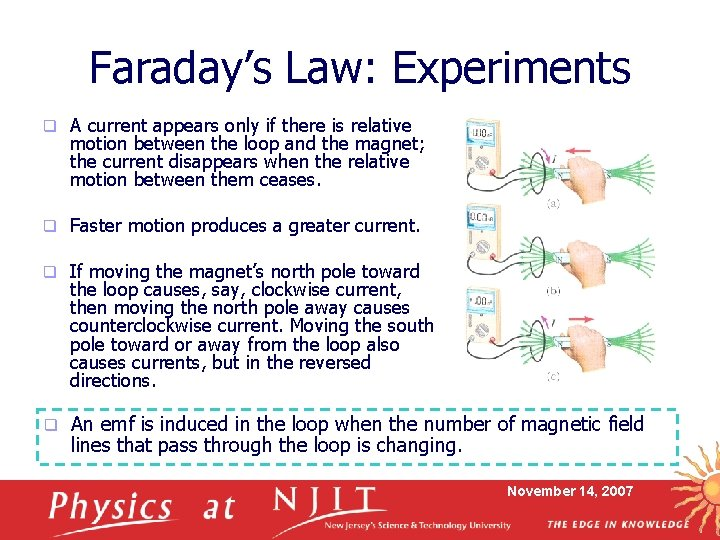Faraday’s Law: Experiments q A current appears only if there is relative motion between the loop and the magnet; the current disappears when the relative motion between them ceases. q Faster motion produces a greater current. q If moving the magnet’s north pole toward the loop causes, say, clockwise current, then moving the north pole away causes counterclockwise current. Moving the south pole toward or away from the loop also causes currents, but in the reversed directions. q An emf is induced in the loop when the number of magnetic field lines that pass through the loop is changing. November 14, 2007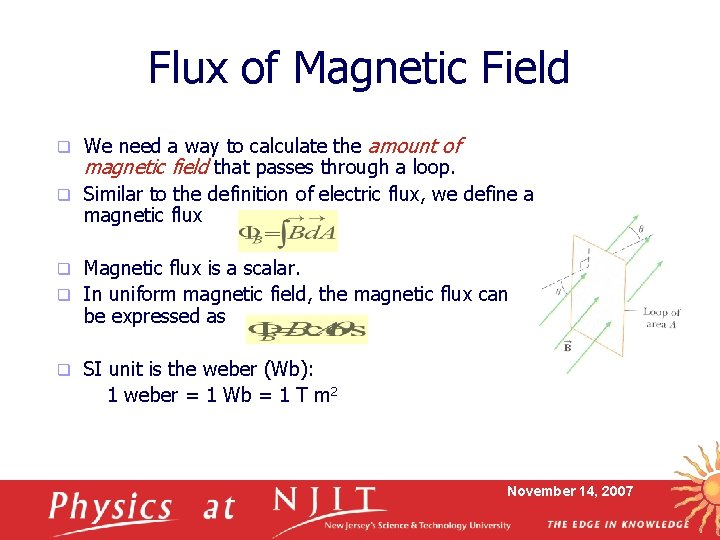Flux of Magnetic Field We need a way to calculate the amount of magnetic field that passes through a loop. q Similar to the definition of electric flux, we define a magnetic flux q Magnetic flux is a scalar. q In uniform magnetic field, the magnetic flux can be expressed as q q SI unit is the weber (Wb): 1 weber = 1 Wb = 1 T m 2 November 14, 2007Faraday’s Law of Induction q q The magnitude of the emf induced in a conducting loop is equal to the rate at which the magnetic flux through that loop changes with time， If a coil consists of N loops with the same area, the total induced emf in the coil is given by q In uniform magnetic field, the induced emf can be expressed as q Emf can be induced in several ways, n n The The Any magnitude of B can change with time. area enclosed by the loop can change with time. angle between B and the normal to the loop can change with time. combination of the above can occur. November 14, 2007Induced Current and Emf 1. A circular loop of wire is held in a uniform magnetic field, with the plane of the loop perpendicular to the field lines. Which of the following will not cause a current to be induced in the loop? A. Pushing the loop into the field. Rotating the loop about an axis perpendicular to the field lines. Keeping the orientation of the loop fixed and moving it along the field lines. B Crushing the loop. Pulling the loop out of the field. B. C. D. E. November 14, 2007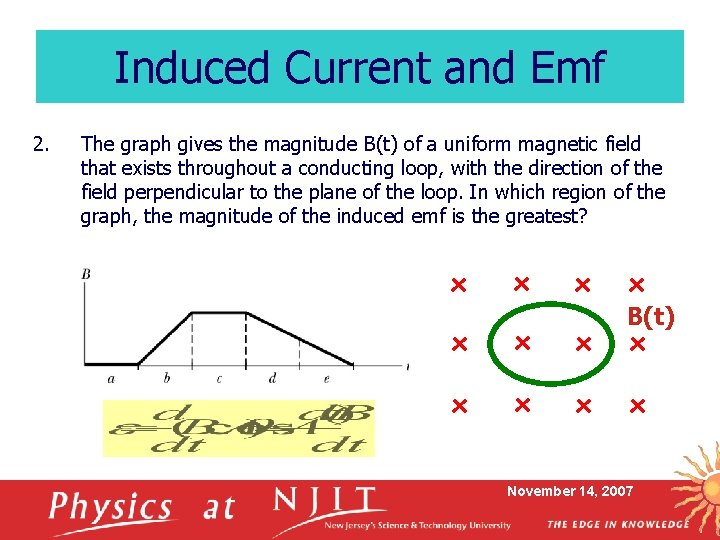Induced Current and Emf 2. The graph gives the magnitude B(t) of a uniform magnetic field that exists throughout a conducting loop, with the direction of the field perpendicular to the plane of the loop. In which region of the graph, the magnitude of the induced emf is the greatest? B(t) November 14, 2007Induction and Energy Transfers A conducting bar of length l sliding along two fixed parallel conducting rails. q Free charges feel a magnetic force along the length of the bar, producing an induced current I. q Start with magnetic flux q Follow Faraday’s law, we have q The change in energy in the system must equal to the transfer of energy into the system by work. q Moving with constant velocity, q q Then q q Power by the applied force is Origin of the induced current and the energy dissipated by the resistor? November 14, 2007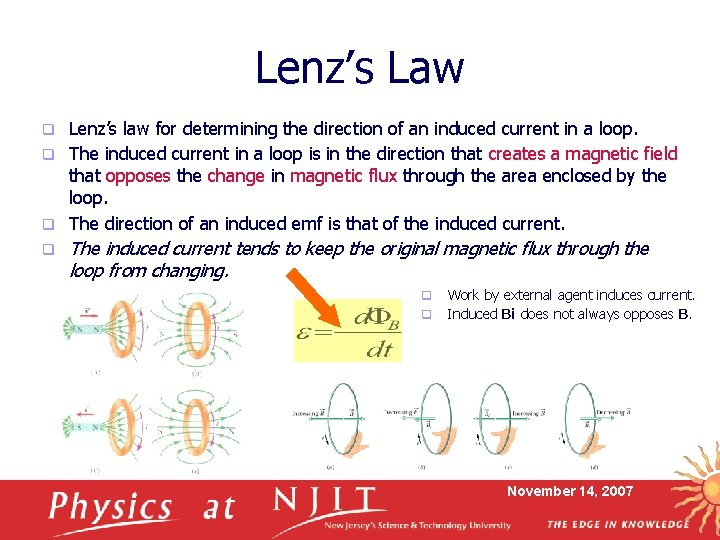Lenz’s Law Lenz’s law for determining the direction of an induced current in a loop. q The induced current in a loop is in the direction that creates a magnetic field that opposes the change in magnetic flux through the area enclosed by the loop. q The direction of an induced emf is that of the induced current. q q The induced current tends to keep the original magnetic flux through the loop from changing. q q Work by external agent induces current. Induced Bi does not always opposes B. November 14, 2007Direction of induced current Which figure is physically reasonable? S E v S S i N N i v N S S N D i S S N N C v N B v=0 S A N 3. i November 14, 2007 SiDirection of induced current 4 A circular loop of wire falling toward a wire carrying a current to the left. What is the direction of the induced current in the loop of wire? A. Clockwise Counterclockwise Zero Impossible to determine B. C. D. v I November 14, 2007A Loop Moving Through a Magnetic Field A rectangular metallic loop of dimensions l and w and resistance R moves with constant speed v to the right. It passes through a uniform magnetic field B directed into the page and extending a distance 3 w along the x axis. Define x as the position of the right side of the loop along the x axis. q Plot as a function of x the magnetic flux, the induced emf, the external applied force necessary to keep v constant. q q Definitions: q Before entering field: Entirely in field: Leaving field: After leaving field: q q November 14, 2007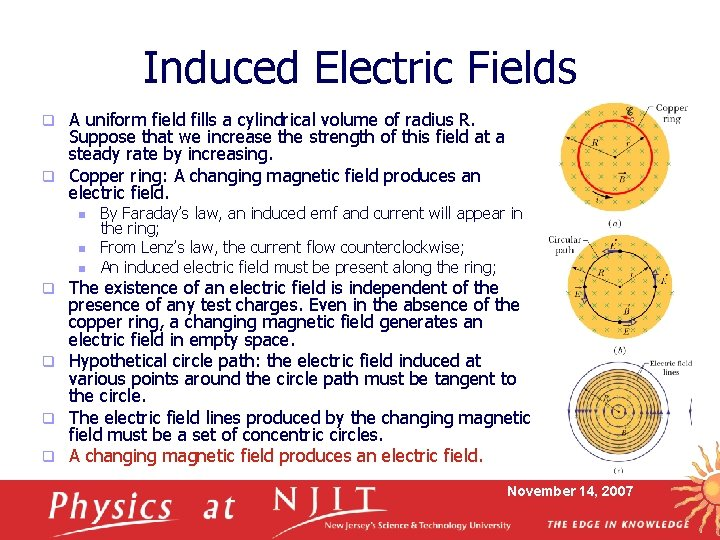Induced Electric Fields A uniform field fills a cylindrical volume of radius R. Suppose that we increase the strength of this field at a steady rate by increasing. q Copper ring: A changing magnetic field produces an electric field. q n n n By Faraday’s law, an induced emf and current will appear in the ring; From Lenz’s law, the current flow counterclockwise; An induced electric field must be present along the ring; The existence of an electric field is independent of the presence of any test charges. Even in the absence of the copper ring, a changing magnetic field generates an electric field in empty space. q Hypothetical circle path: the electric field induced at various points around the circle path must be tangent to the circle. q The electric field lines produced by the changing magnetic field must be a set of concentric circles. q A changing magnetic field produces an electric field. q November 14, 2007A Reformulation of Faraday’s Law q q A charge q 0 moving around the circular path. The work W done by the induced electric field, q The work done in moving the test charge around the path, q Two expressions for W equal to each other, we find, q A more general expression for the work done on a charge q 0 moving along any closed path, q So, q Combined with Faraday’s law, q Electric potential has meaning only for electric fields produced by static charges; it has no meaning for that by induction. November 14, 2007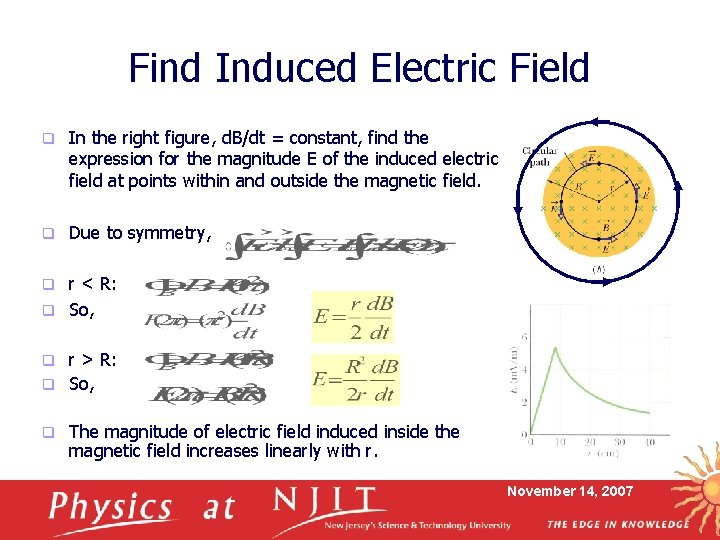Find Induced Electric Field q In the right figure, d. B/dt = constant, find the expression for the magnitude E of the induced electric field at points within and outside the magnetic field. q Due to symmetry, r < R: q So, q r > R: q So, q q The magnitude of electric field induced inside the magnetic field increases linearly with r. November 14, 2007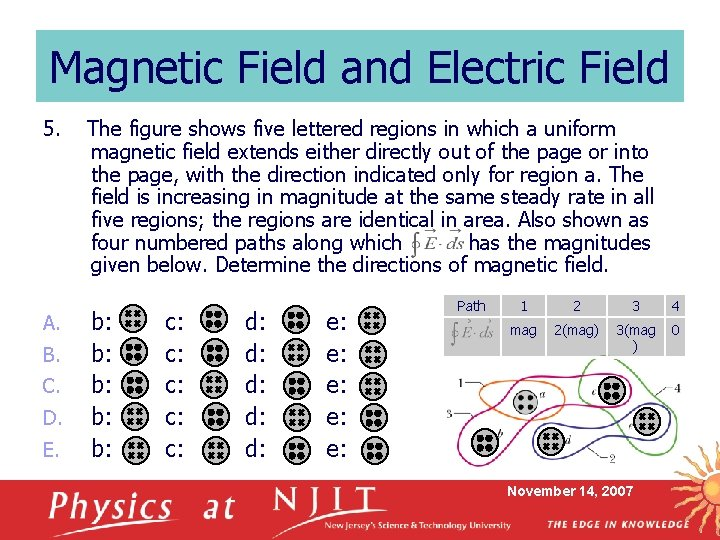Magnetic Field and Electric Field 5. A. B. C. D. E. The figure shows five lettered regions in which a uniform magnetic field extends either directly out of the page or into the page, with the direction indicated only for region a. The field is increasing in magnitude at the same steady rate in all five regions; the regions are identical in area. Also shown as four numbered paths along which has the magnitudes given below. Determine the directions of magnetic field. b: b: b: c: c: c: d: d: d: e: e: e: Path 1 2 3 4 mag 2(mag) 3(mag ) 0 November 14, 2007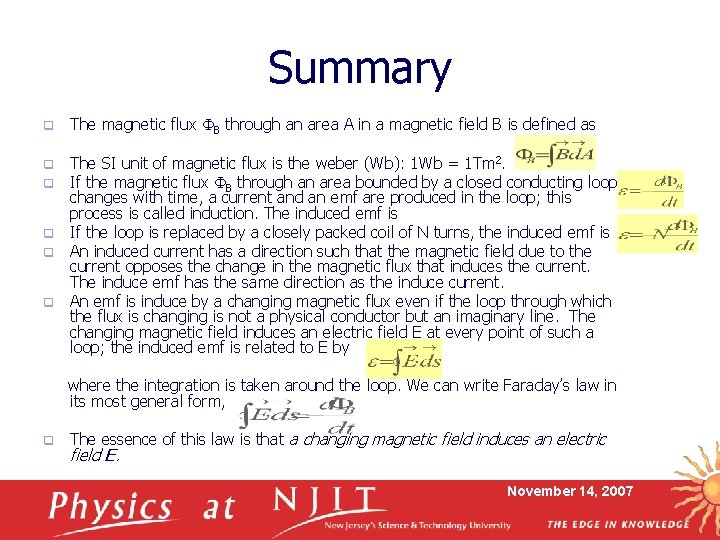Summary q The magnetic flux B through an area A in a magnetic field B is defined as The SI unit of magnetic flux is the weber (Wb): 1 Wb = 1 Tm 2. If the magnetic flux B through an area bounded by a closed conducting loop changes with time, a current and an emf are produced in the loop; this process is called induction. The induced emf is q If the loop is replaced by a closely packed coil of N turns, the induced emf is q An induced current has a direction such that the magnetic field due to the current opposes the change in the magnetic flux that induces the current. The induce emf has the same direction as the induce current. q An emf is induce by a changing magnetic flux even if the loop through which the flux is changing is not a physical conductor but an imaginary line. The changing magnetic field induces an electric field E at every point of such a loop; the induced emf is related to E by q q where the integration is taken around the loop. We can write Faraday’s law in its most general form, q The essence of this law is that a changing magnetic field induces an electric field E. November 14, 2007